## Bearing Strength of Soil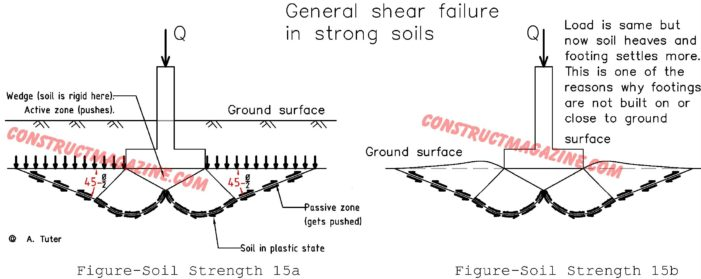So far, under soil strength section, we have explained total and effective stresses, pore pressure, angle of internal friction, shear strength, lateral earth pressure coefficient, and cohesion of clay. Now we are ready to start seeing how these are actually used, when designing foundations.   In this subsection we will now learn about bearing strength, […]

## Cohesion of Clay

Cohesion, although thought to have only one meaning by many engineers, actually has two close meanings to each other. One meaning is in soil physics, which means, is the attraction of particles in soil, creating a sticking action. The other meaning comes for soil mechanics, and it means the shear strength of soil, when there […]

## Angle of repose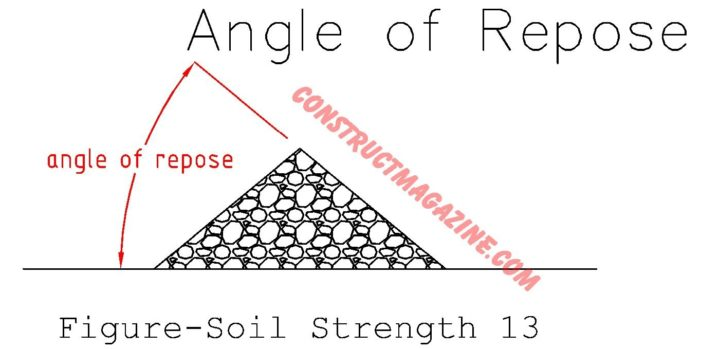We talked about angle of internal friction of soil, in previous posts of this series. There is also the angle of repose, which is often confused with angle of internal friction. Angle of repose is the angle a soil forms with the horizontal, when poured over a surface in it’s loosest state. Although angle of […]

## Triaxial Test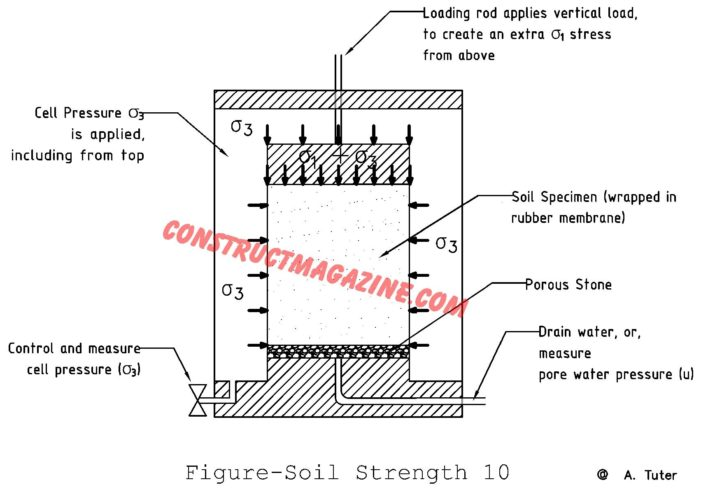A more complicated version of direct shear test which we talked about in the previous post, is called triaxial test, where you can also control the water drainage turning off or on, plus you can measure at what angle the sample will shear, which we will talk about more in the following paragraphs. Both of […]

## Direct Shear test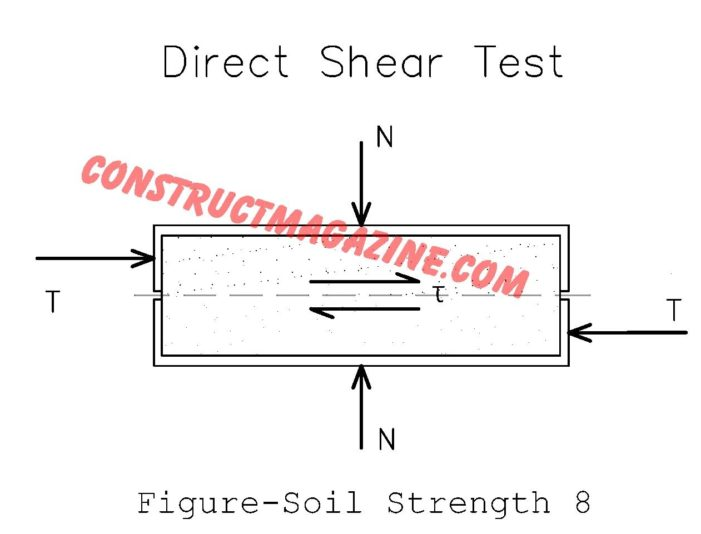When we want to test the shear strength of soil vs. how much we press on it vertically, we can use a mechanism like below, which is called direct shear test. It is the most basic shear test method for soil. In it’s simplest from it can be shown as: Direct shear test is a […]

## Angle of Internal Friction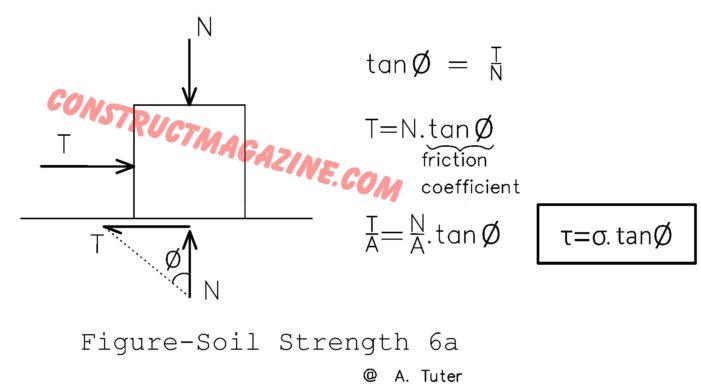Think of this post as a direct continuation of the previous two posts of this series, shear strength of soil and lateral earth pressure. Now let’s present another very important characteristic of soil, the angle of internal friction, which we briefly mentioned already before in those sections. Let’s consider a block resting on a surface, […]

## Example for Lateral Earth Pressure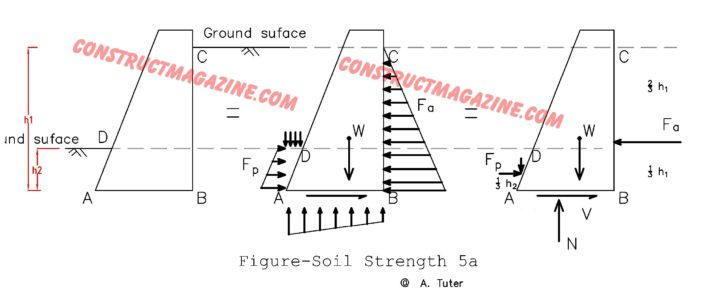Now let’s give a simple example to wrap things up. This example will use many things we have learned so far, not only in soil mechanics, but structural basics section too. We have a gravity retaining wall here, which is a type of retaining wall that resists overturning effect of retained soil by its weight. […]

## Lateral Earth Pressure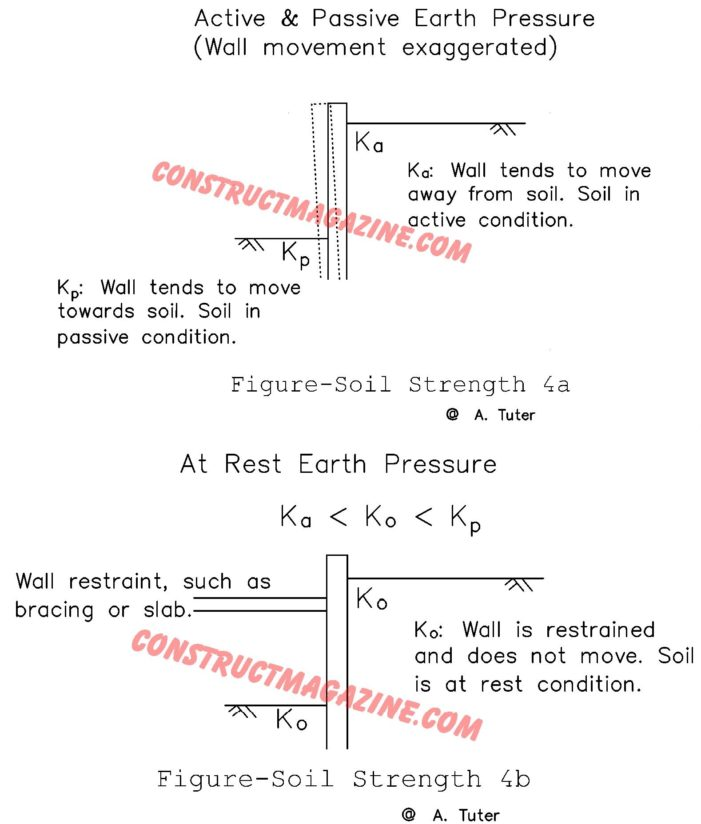In designing retaining structures, the most critical thing to know is how much horizontal (lateral) pressure the retained soil will exert on the retaining structure, such as a retaining wall. In previous post, we learned how to calculate the vertical stresses soils. Here, we must somehow derive horizontal pressure from it. To do this, we […]

## Shear Strength of Soil

We introduced shear stress before in this book. If you are not an engineer, and forgot what shear stress means, first please go back to section Structural Basics > Forces and look at again, what it means “shear”. Now, Imagine the following different situations: Think of water running down a sloped street. It can flow, […]

## Total Stress, Effective Stress, Pore Water Pressure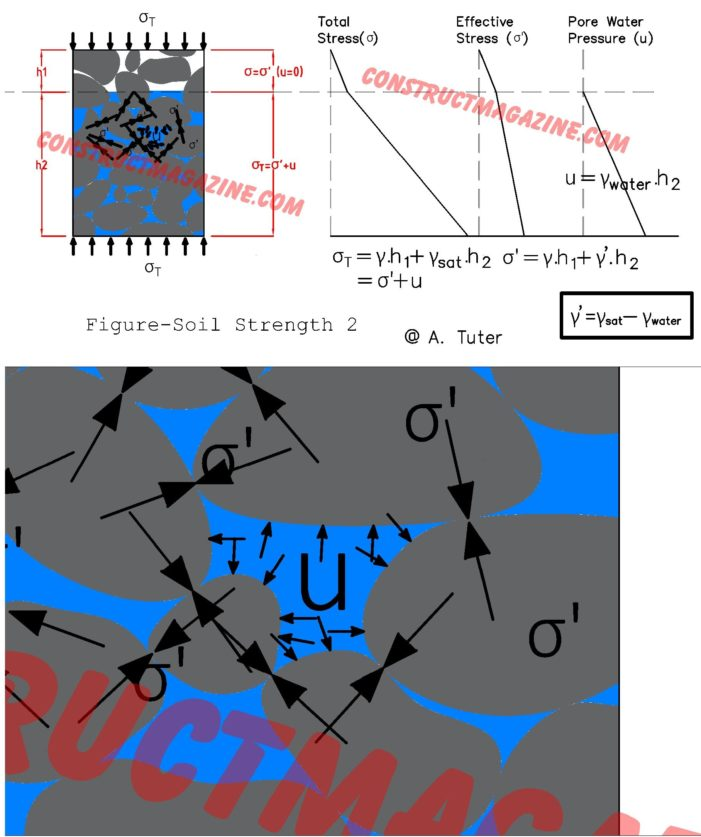Before we move on here with soils, let’s talk about fluid pressure first. The pressure of any fluid (liquid or gas), such as water, increases constantly with depth such as: Pressure = Unit Weight of the Material x Depth Written in correct terms: Pressure = γfluid x d, where, γ: the unit weight of the […]